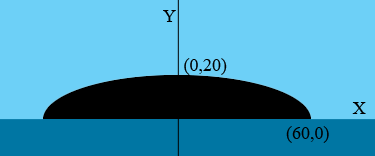SEARCH HOMEMath Central Quandaries & QueriesQuestion from Ian a student: The arch of the bridge is in the shape of semi ellipse,with its major axis at the water level.suppose the arch is 20ft. High in the middle,and 120 ft. Across its major axis. How high above the water level is the arch,at a point 20 ft.from the center (horizontally). Round of 2 decimal placesHi Ian,

I drew a diagram with the X-axis at the water and the middle of the bridge at the Y-axis.Since the arch of the bridge is in the shape of a semi ellipse the equation of the ellipse is

$\frac{x^2}{a^2} + \frac{y^2}{b^2} = 1$

for some real numbers $a$ and $b.$

Use the co-ordinates of two of the points you know on the bridge to find $a$ and $b.$

PennyMath Central is supported by the University of Regina and The Pacific Institute for the Mathematical Sciences.